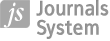PRACA ORYGINALNA
Reguły polityki monetarnej w warunkach długookresowej równowagi typu Domara-Solowa

Więcej
Ukryj
Data publikacji: 25-09-2003

GNPJE 2003;186(9):1–21

STRESZCZENIE
This study provides an analysis of monetary policy rules base on the Domar-Solow growth model. The study adopts the following assumptions: 1. Investment affects both the demand and the supply-side of the economy. Thanks to multiplier effects, the rise in investment involves demand growth (the Domar model), and through capital formation it adds to aggregate supply (Domar and Solow models). 2. Investment is a decreasing function of the interest rate, which is set by the central bank. 3. Supply is generated by the neoclassical production function (the Solow model). 4. The central bank sets interest rates at such a level so that (i) demand equalled supply, thus leading to full capacity utilisation in the economy; (ii) the unemployment rate was stable. The main conclusions to be drawn from the above model are the following: 1. The capital growth rate is described by the Riccati Differential Equation, which provides a stable long-term solution. 2. Growth rates of interest rates should be decreasing, linear functions of the growth rate of capital and should be (generally) negative. 3. The model excludes the so-called Domar paradox involved with the "knife-edge" issue due to the flexible capital-intensity ratio represented in the function. 4. Long-term capital and GDP growth rates are lower than is the case with the Solow growth model.
Udostępnij
 eISSN: 2300-5238